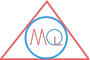# Area and Perimeter of Circle

## Introduction

The very common measurements that can we do for a circle are circumference of circle, area of circle, area of sector, length of arc and area of segment.

So, let’s discuss about them and see how to calculate them.

## Circumference of circle

Circumference is distance around the circle. Its units are same as that of length i.e meter, centimeter, millimeter etc.

We can calculate the circumference of circle using the formula as below:

Formula

Circumference of circle = $$2 \pi r$$

where, $$\pi$$ is a constant value and r is radius of circle

The constant value of $$\pi = \frac{22}{7}$$ or $$3.14$$

Example

Calculate circumference of circle whose radius is 5cm.

Here, radius of circle = 5cm

As we know, Circumference of circle = $$2 \pi r$$

$$\therefore$$, Circumference of circle = $$2 \pi \times 5$$

$$= 2 \times 3.14 \times 5$$

$$= 31.4 \; cm$$

Note

What is $$\pi$$?

$$\pi$$ is a value that never changes. If calculated, it always remains constant i.e. $$3.14$$.

How is it calculated?

It is calculated by dividing the circumference of circle to diameter of circle.

It is a ratio of circumference and diameter of circle.

This constant value is denoted by $$\pi$$ and read as pi.

$$\pi = \frac{Circumference}{Diameter}$$

## Area of circle

Area of circle is a space occupied by a circle. Its units are i.e $$meter^2$$, $$centimeter^2$$, $$millimeter^2$$ etc.

Formula

Area of circle = $$\pi r^2$$

where, $$\pi$$ is a constant value and r is radius of circle

Again, the constant value of $$\pi = \frac{22}{7}$$ or $$3.14$$

Example

Calculate area of circle whose radius is 10cm.

Here, radius of circle = 10cm

As we know, Area of circle = $$\pi r^2$$

$$\therefore$$, Area of circle = $$\pi \times 10^2$$

$$= 3.14 \times 100$$

$$= 314 \; cm^2$$

For the following below measurements, please refer to this figure.

Sector area of circle## Area of sector

Formula

Area of sector of angle $$\theta$$ = $$\frac{\theta}{360} \times \pi r^2$$

### Area of major sector

Formula

Area of major sector OADB = $$\pi r^2$$ – Area of minor sector OACB

## Length of arc

Formula

Length of arc of sector of angle $$\theta$$ = $$\frac{\theta}{360} \times 2 \pi r$$

## Area of segment

Formula

Area of segment ACB = $$\pi r^2$$ – Area of triangle

### Area of major segment

Formula

Area of major segment ADB = $$\pi r^2$$ – Area of minor segment ACB